Open In App
Related Articles

# Height and Distance

Heights and Distances is the real-life application of trigonometry Trigonometry is useful to astronomers, navigators, architects, surveyors, etc. in solving problems related to heights and distances. In height and distance, we use trigonometric concepts to find the height and distance of various objects.

## What Do You Mean by Height and Distance?

Various terminologies which are helpful in understanding Height and Distance are,

• Line of sight: It is the line drawn from the eye of an observer to the point in the object viewed by the observer.
• Angle of Elevation: The angle between the horizontal and the line of sight joining an observation point to an elevated object is called the angle of elevation.
• Angle of depression: The angle between the horizontal and the line of sight joining an observation point to an object below the horizontal level is called the angle of depression.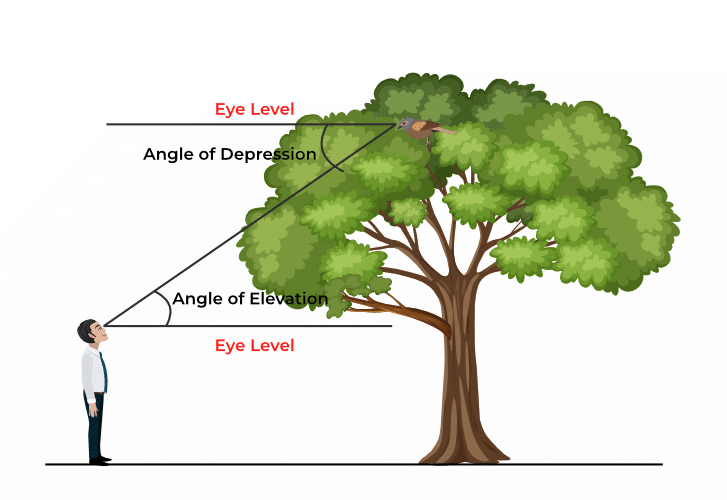## How to Find Heights and Distances?

Trigonometric ratios are used to measure the heights and distances of different objects. For example, in the above figure, a person is looking at the top of the tree the angle from the eye level to the top of the tree is called the angle of elevation and similarly, the angle from the top of the tree to the eyes of the person is called the angle of depression. If the height of the person and its distance from the tree is known we can easily calculate the height of the tree.

## Trigonometric Ratios Table

The value of trigonometric ratios for different angles is very useful for solving Height and Distance problems. Thus it is advised to learn the values of trigonometric ratios for different angles. The value of various trigonometric ratios can be learned using the trigonometric table provided below,

## Solved Examples on Height and Distance

Example 1: If a pole 6 m high casts a shadow 2√3 m long on the ground, find the Sun’s elevation.

Solution: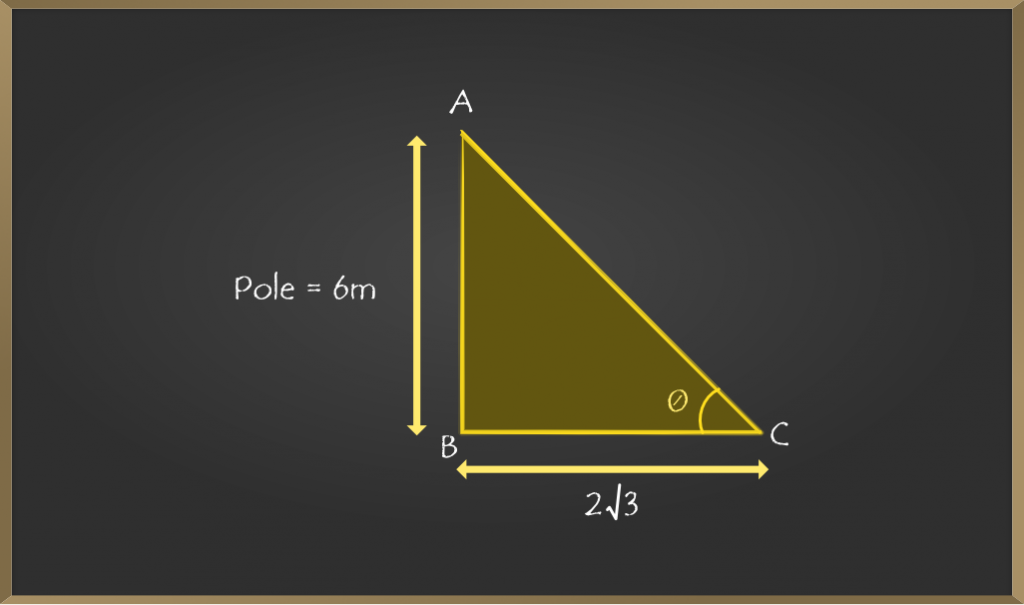Let AB be the pole which is of height 6 m.

Let BC be the shadow of the building 2√3.

Now, in ∆ ABC,

tan θ = AB / BC

tan θ = 6 / 2√3

Now, simplifying using rationalization

tan θ = (3 / √3)*(√3 / √3)

tan θ = 1 / √3

θ = tan-1(1 / √3)

Hence, θ = 60o

Therefore, sun’s elevation from the ground is 60o.

Example 2: An observer 1.5 m tall is 20.5 m away from a tower 22 m high. Determine the angle of elevation of the top of the tower from the eye of the observer.

Solution: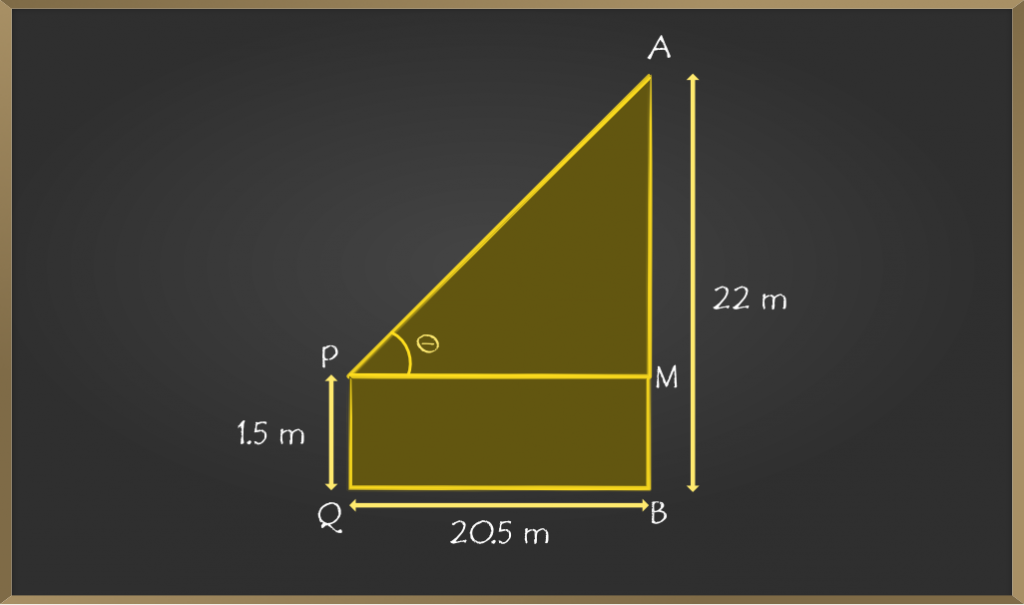Let PQ be the height of the observer of 1.5 m.

Let AB be the height of the tower of 22 m.

And, let QB be the horizontal distance between the observer and the tower

PQ = MB = 1.5 m

AM = AB – MB

AM = 22 – 1.5 = 20.5

Now, in ∆APM,

tan θ = AM / PM

tan θ = 20.5 / 20.5

tan θ = 1

θ = tan-1(1 )

Hence, θ = 45o

Therefore, the angle of elevation of the top of the tower from the eye of the observer is 45o

Example 3: An aeroplane is flying h meters above the ground. At a particular instant, the angle of elevation of the plane from the eyes of a boy sitting on the ground is 60°. After some time, the angle of elevation changed to 30°. Find the distance covered by the plane during that time assuming it travelled in a straight line.

Solution: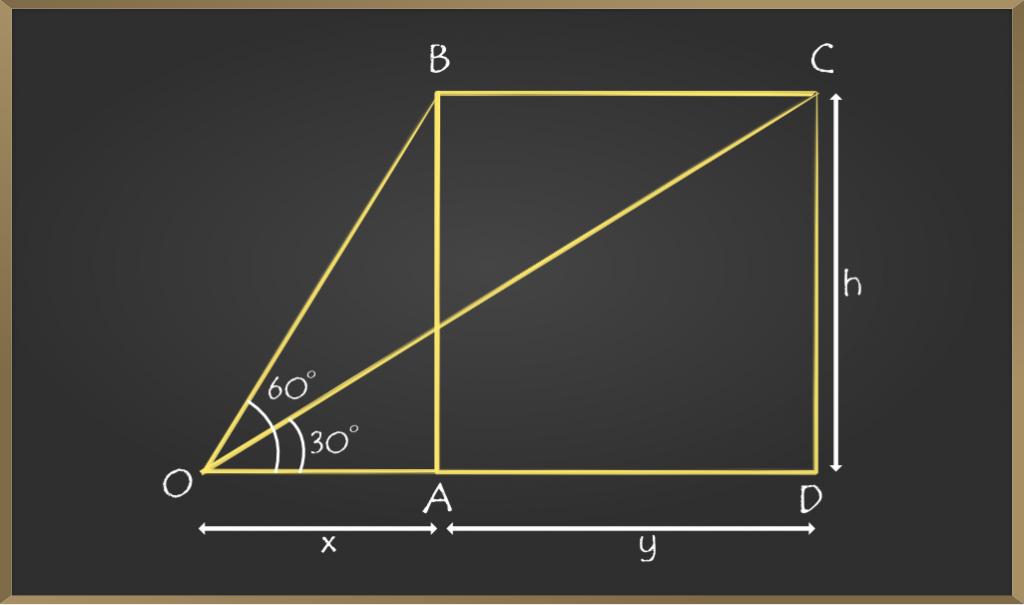Let x be the horizontal distance between the observer and plane at the first instant.

Let y be the horizontal distance between the observer and plane at the second instant.

And, BA = CD = h

In ∆OAB,

tan 60° = AB / OA

√3 = h / x

x = h / √3

In ∆ OCD,

tan 30° = CD / OD

1/√3 = h / (x+y)

x + y = √3h

Distance travelled by plane = AD = y

(x + y) − x = √3h − h / √3

y = (2 / √3)h

So, if the airplane is flying h meters above the ground, it would travel for (2/√3) h meters as the angle of elevation changes from 60° to 30°.

Example 4: From the top of the tower 30 m height a man is observing the base of a tree at an angle of depression measuring 30 degrees. Find the distance between the tree and the tower.

Solution: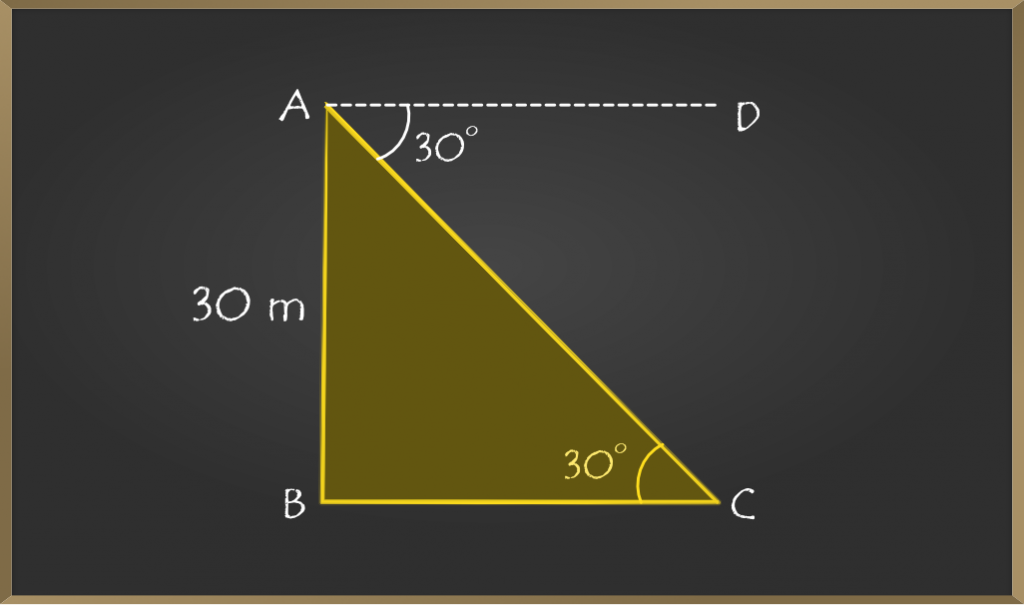In the above diagram AB represents the height of the tower, BC represents the distance between the foot of the tower and the foot of the tree.

Now we need to find the distance between the foot of the tower and the foot of the tree (BC). For that as angle of depression is given so by vertically opposite angle property of triangle ∠CAD = ∠BCA

In ∆BCA,

tan θ = Opposite side / Adjacent side

tan 30° = AB / BC

1/√3 = 30 / BC

BC = 30√3

BC = 30 (1.732)      [Approximately]

BC = 51.96 m

So, the distance between the tree and the tower is 51.96 m.

Example 5: From the top of a building 30 m high, the top and bottom of a tower are observed to have angles of depression 30° and 45° respectively. Find the height of the tower.

Solution: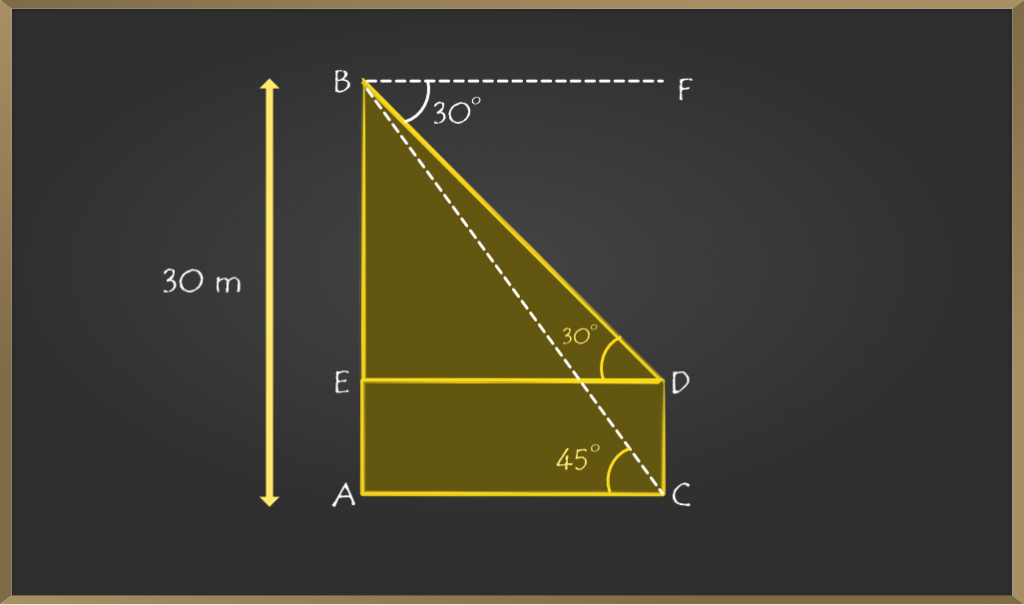Let AB be the building and CD be the tower.

The angle of depressions is given 30° and 45° to the top and bottom of the tower. So by vertically opposite triangle property ∠FBD = ∠EDB and ∠FBC = ∠ACB.

Now, AB = 30 m. Let DC = x.

Draw DE perpendicular AB. Then AE = CD = x.

Therefore  BE = (30 – x) m.

In ∆ACB,

cot θ = Adjacent side / Opposite side

cot θ = AC / AB

cot 45° = AC / 30

AC = 30              [cot 45° = 1]

So, DE = AC = 30 m

In ∆EDB,

tan θ = Opposite side / Adjacent side

tan 30° = BE / DE

1/√3 = BE / 30

BE = 30 / √3

CD = AE = AB – BE = 30 – (30 / √3)

30[1 – (1 / √3) ] m

Height of the tower is 30[1 – (1 / √3) ] m

## FAQs on Height and Distances

### Question 1: What is the angle of depression in trigonometry?

The angle of depression is defined as the angle between the observer’s horizontal line of sight and the object when the observer is looking at the object which is placed downward.

### Question 2: What is the angle of elevation in trigonometry?

The angle of elevation is defined as the angle between the observer’s horizontal line of sight and the object when the observer is looking at the object which is placed upward.